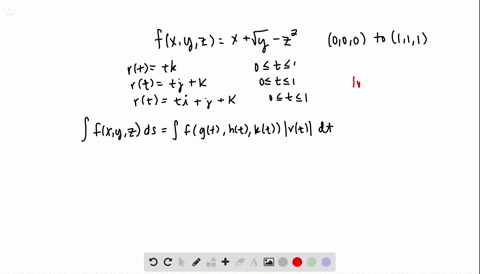🎉 The Study-to-Win Winning Ticket number has been announced! Go to your Tickets dashboard to see if you won! 🎉View Winning Ticket### Integrate $f(x, y, z)=x+\sqrt{y}-z^{2}$ over the …

08:37Oklahoma Baptist University
Problem 15

# Integrate $f(x, y, z)=x+\sqrt{y}-z^{2}$ over the path from $(0,0,0)$ to $(1,1,1)$ ( see accompanying figure) given by$$C_{1} : \quad \mathbf{r}(t)=t \mathbf{i}+t^{2} \mathbf{j}, \quad 0 \leq t \leq 1$$$$C_{2} : \quad \mathbf{r}(t)=\mathbf{i}+\mathbf{j}+t \mathbf{k}, \quad 0 \leq t \leq 1$$

## Discussion

You must be signed in to discuss.